# KSEEB Solutions for Class 9 Maths Chapter 7 Quadrilaterals Ex 7.1

KSEEB Solutions for Class 9 Maths Chapter 7 Quadrilaterals Ex 7.1 are part of KSEEB Solutions for Class 9 Maths. Here we have given Karnataka Board Class 9 Maths Chapter 7 Quadrilaterals Exercise 7.1.

## Karnataka Board Class 9 Maths Chapter 7 Quadrilaterals Ex 7.1

Question 1.
The angles of a quadrilateral are in the ratio 3 : 5 : 9 : 13. Find all the angles of the quadrilateral.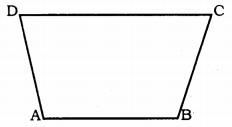Solution:
The angles of a quadrilateral are in the ratio 3 : 5 : 9 : 13.
Let ∠A : ∠B : ∠C : ∠D = 3 : 5 : 9 : 13.
Sum of ratio = 3x + 5x + 9x + 13x = 30x
Sum of 4 angles of quadrilateral is 360°
∴ ∠A + ∠B + ∠C + ∠D = 360°
3x + 5x + 9x + 13x = 360
30x = 360
∴ x = $$\frac{360}{30}$$ = 12°
∠A = 3x = 3 × 12 = 36°
∠B = 5x = 5 × 12 = 60°
∠C = 9x = 9 × 12 = 108°
∠D = 13x = 13 × 12 = 156°

Question 2.
If the diagonals of a parallelogram are equal, then show that it is a rectangle.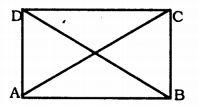Solution:
Data: Diagonals of a parallelogram are equal.
To Prove: ABCD is a rectangle.
Proof: Now ABCD is a parallelogram and diagonal AC = Diagonal BD (Data)
In ∆ABC and ∆ABD,
AC = BD (Data)
AB common.
∴ ∆ABC ≅ ∆ABD (SSS postulate)
But, ∠ABC + ∠BAD = 180°
∠ABC + ∠ABC = 180°
2 ∠ABC = 180°
∴ ∠ABC = 90°
If an angle of a parallelogram is a right angle, it is called a rectangle.
∴ ABCD is a rectangle.

Question 3.
Show that if the diagonals of a quadrilateral bisect each other at right angles, then it is a rhombus.Solution:
Data: ABCD is a parallelogram and diagonals AC and BD bisect at right angles at O’.
To Prove: ABCD is a rhombus.
Proof: Here, AC and BD bisect each other at right angles.
∴ AO = OC
BO = OD
and ∠AOB = ∠BOC = ∠COD = ∠AOD = 90°
If sides are equal to each other, then ABCD is said to be a rhombus.
Now, ∆AOD and ∆COD,
AO = OC (Data)
∠AOD = ∠COD = 90° (Data)
OD is common.
∴ ∆AOD ≅ ∆COD (SAS Postulate)
∴ AD = CD …………… (i)
Similarly,
∆AOD = ∆AOB
∆AOB ≅ ∆COB
∴ AB = BC ……….. (iii)
∆COB ≅ ∆COD
∴ BC = CD ……………. (iv)
From (i), (ii), (iii) and (iv),
AB = BC = CD = AD
All 4 sides of parallelogram ABCD are equal, then it is a rhombus.

Question 4.
Show that the diagonals of a square are equal and bisect each other at right angles.Solution:
Data: ABCD is a parallelogram. Its diagonals are AC and BD. They meet at ‘O’.
To Prove: i) AO = OC
BO = OD
ii) ∠AOB = ∠BOC = ∠COD = ∠DOA = 90°.
Proof: In ∆ABC and ∆ABD,
BC = AD (diagonals of square is equal)
∠ABC = ∠BAD = 90° (angles of square)
AB is common.
∴ ∆ABC ≅ ∆ABD (SAS Postulate.)
In ∆AOB and ∆COD,
AB = DC (sides of square)
∠OAB = ∠OCD (alternate angles)
∠OBA = ∠ODC (alternate angles)
∴ ∆AOB ≅ ∆COD (ASA Postulate)
AO = OC
BO = OD …………….. (i)
Similarly,
∆AOB ≅ ∆BOC.
∴ ∠AOB = ∠BOC = 90°
Now, ∆COD ≅ ∆AOD, then
∴ ∠COD = ∠DOA = 90°
∴ ∠AOB = ∠BOC = ∠COD = ∠DOA = 90° …………. (ii)
from (i) and (ii)
∴ Sides of a square are equal and bisect at right angles.

Question 5.
Show that if the diagonals of a quadrilateral are equal and bisect each other at right angles, then it is a square.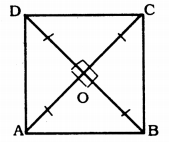Solution:
Data: ABCD is a quadrilateral and bisects each other at right angles, then it is a square.
AO = OC and AC = BD
BO = OD
∠AOB = ∠BOC = ∠COD + ∠DOA = 90°.
To Prove: ABCD is a square.
Proof: In ∆AOB and ∆COD,
AO = OC
BO = OD (Data)
∠AOB = ∠COD (Vertically opposite angles)
∴ ∆AOB ≅ ∆COD (SAS Postulate)
AB = CD …………. (i)
∠ABO = ∠CDO
∴ AB || CD ………… (ii)
From (i) and (ii) ABCD is a parallelogram.
Now, in ∆AOD and ∆COD,
AO = OC (Data)
∠AOD = ∠COD = 90° (Data)
OD is common
∴ ∆AOD ≅ ∆COD (SAS Postulate)
From (ii), (iii) and (iv)
AB = BC = CD = AD
Four sides of a quadrilateral are they are equal to each other and bisect each other at right angles, then it is a square.
∴ ABCD is a square.

Question 6.
Diagonal AC of a parallelogram ABCD bisects ∠A. Show that(i) it bisects ∠C also,
(ii) ABCD is a rhombus.
Solution:
Data: Diagonal AC of a parallelogram ABCD bisects ∠A.
To Prove: (i) Diagonal AC also bisects ∠C.
(ii) ABCD is a rhombus.
Proof: i) Diagonal AC also bisects ∠A.
∴ ∠DAC = ∠BAC …………… (i)
But, ∠DAC= ∠BCA (alternate angles)(ii)
∠BAC=∠DCA (alternate angles)(iii)
From (i), (ii) and (iii),
∠BCA = ∠DCA
∴ AC bisects ∠C.

(ii) ∠DAC = ∠DCA
∴ DC = AB
∴ AB = BC = CD = DA
∴ ABCD is a rhombus.

Question 7.
ABCD is a rhombus. Show that diagonal AC bisects ∠A as well as ∠C and diagonal BD bisects ∠B as well as ∠D.Solution:
ABCD is rhombus. Diagonal AC bisects ∠A and ∠C.
To Prove: Diagonal BD bisects ∠B and ∠D.
Proof: In a rhombus all sides are equal and opposite angles are equal to each other.
In ∆ABC,
AB = BC
∴ ∠BAC = ∠BCA
∠B AC = ∠DCA (alternate angles)
∴ ∠BCA = ∠DCA …………… (i)
∴ AC bisects ∠C.
Now, ∠BCA = ∠DAC (alternate angles)
∠BAC = ∠DAC
∴ AC bisects ∠A
In ∆ABD,
∠ABD = ∠CDB
∴ BD bisects ∠D.
∠ABD = ∠CBD
∴ BD bisects ∠B.

Question 8.
ABCD is a rectangle in which diagonal(i) ABCD is a square,
(ii) Diagonal BD bisects ∠B as well as ∠D.
Solution:
Data: ABCD is a rectangle in which diagonal AC bisects ∠A as well as ∠C.
To Prove:
(i) ABCD is a square.
(ii) Diagonal BD bisects ∠B as well as ∠D.
Proof: (i) ABCD is a rectangle. AC and BD diagonals intersect at O’.
AB = DC (Opposite sides of rectangle)
∴ $$\frac{1}{2}$$ ∠BAD = $$\frac{1}{2}$$ ∠BCD
∴ ∠DAC = ∠DCA (AC bisects ∠A)
∴ DC = DA
But, AB = CD and DA = BC (Data)
∴ AB = BC = CD = AD
∴ ABCD is a square.

(ii) In ∆ABD.
∠ABD = ∠CDB (alternate angles)
∴ BD bisects ∠D.
∠ABD = ∠CBD (alternate angles)
∴ BD bisects ∠B.

Question 9.
In parallelogram ABCD. two points P and Q are taken on diagonal BD such that DP = BQ. Show that: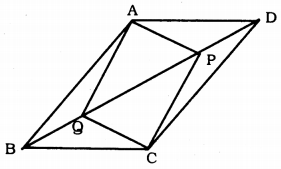(i) ∆APD ≅ ∆CQB
(ii) AP = CQ
(iii) ∆AQB ≅ ∆CPD
(iv) AQ = CP
(v) APCQ is a parallelogram.
Solution:
Data: In parallelogram ABCD, two points P and Q are taken on diagonal BD such that DP = BQ.
To Prove:
(i) ∆AAPD ≅ ∆CQB
(ii) AP = CQ
(iii) ∆AQB ≅ ∆CPD
(iv) AQ = CP
(v) APCQ is a parallelogram.
Proof: ABCD is a parallelogram.
(i) In ∆APD and ∆CQB,
DP = BQ (Data)
∴ ∆APD ≅ ∆CQB (ASA Postulate)

(ii) AP = CQ

(iii) In ∆AQB and ∆CPD,
AB = CD (opposite sides)
∠ABQ = ∠CDP (alternate angles)
BQ = DP (Data)
∴ ∆AQB ≅ ∆CPD (ASA Postulate)

(iv) ∴ AQ = CP

(v) In ∆AQPand ∆CPQ,
AP = CQ (proved)
AQ = PC PQ is common.
∴ ∆AQP ≅ ∆CPQ
∴ ∠APQ = ∠CQP
These are a pair of alternate angles.
∴AP || PC
Similarly, AQ || PC
∴ APCQ is a parallelogram.

Question 10.
ABCD is a parallelogram and AP and CQ are perpendiculars from vertices A and C on diagonal BD. Show that(i) ∆APB ≅ ∆CQD
(ii) AP = CQ.
Solution:
Data: ABCD is a parallelogram and AP and CQ are perpendiculars from vertices A and C on diagonal BD.
To Prove:
(i) ∆APB ≅ ∆CQD
(ii) AP = CQ.
Proof: ABCD is a parallelogram. BD is diagonal.
AP ⊥ BD and CQ ⊥ BD.

(i) In ∆APB and ∆CQD,
∠APB = ∠CQD = 90°
AB = CD (opposite sides)
∠ABP = ∠CQD (alternate angles)
∴ ∆APB ≅ ∆CQD (AAS postulate)

(ii) ∴ AP = CQ.

Question 11.
In ∆ABC and ∆DEF, AB = DE, AB || DE, BC = EF and BC || EF. Vertices A, B, and C are joined to vertices D. E and F respectively. Show that(i) Quadrilateral ABED is a parallelogram.
(ii) Quadrilateral BEFC is a parallelogram.
(iv) quadrilateral ACFD is a parallelogram.
(v) AC = DF
(vi) ∆ABC ≅ ∆DEF.
Solution:
Data: In ∆ABC and ∆DEF, AB = DE, AB || DE, BC = EF and BC || EF.
Vertices A, B, and C are joined to vertices D, E, and F respectively.
To Prove:
(i) Quadrilateral ABED is a parallelogram.
(ii) Quadrilateral BEFC is a parallelogram.
(iv) quadrilateral ACFD is a parallelogram.
(v) AC = DF
(vi) ∆ABC ≅ ∆DEF.
Proof: (i) AB = DE and AB||DE (Data)
∴ ABCD is a parallelogram.

(ii) Similarly, BC = EF and BC || EF.
∴ BE = CF
BE || CF
∴ BEFC is a parallelogram.

(iii) ABED is a parallelogram.
Similarly, BEFC is a parallelogram.
∴ CF = BE
CF || BE ……………. (ii)
Comparing (i) and (ii),

∴ AC = DF
AC || DF
∴ ACFD is a parallelogram.

(v) ACFD is a parallelogram.
AC = DF (opposite sides).

(vi) In ∆ABC and ∆DEF,
AB = DE (Data)
BC = EF (opposite sides of a
parallelogram)
AC = DF (Opposite sides of a
parallelogram)
∴ ∆ABC ≅ ∆DEF (SSS postulate).

Question 12.
ABCD is a trapezium in which AB||CD and AD = BC . Show that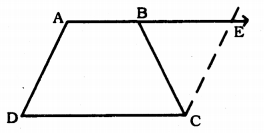(i) ∠A = ∠B
(ii) ∠C = ∠D
(iv) diagonal AC = diagonal BD.
(Hint: Extend AB and draw a line through C parallel to DA intersecting AB produced at E.]
Solution:
Data: ABCD is a trapezium in which AB || CD and AD = BC.
To Prove:
(i) ∠A = ∠B
(ii) ∠C = ∠D
(iv) diagonal AC = diagonal BD.
Construction: Produce straight line AB.
Draw a parallel line DA, parallel to AB such that the produced line meet this at E.
Proof: (i) ABCD is a trapezium.
AB || CD and AD = BC (Data)
Now, AD || CD and AB || DC.
∴ AD = CE (Opposite sides)
CE = BC
∴ ∠CBE = ∠CEB
Now, ∠DAB + ∠CEB = 180° (Sum of consecutive angles.)
∴ ∠DAB + ∠CEB = 180°
( ∵ ∠CEB = ∠CBE) ……………… (i)
Now, ∠ABC + ∠CDE = 180°
(linear pair) ……………….. (ii)
Comparing (i) and (ii),
∠DAB + ∠CBE = ∠ABC + ∠CBE
∴ ∠DAB = ∠ABC Or ∠A = ∠B.

(ii) AB || CD
∠DAB + ∠ADC =180° ……….. (iii)
∠ABC + ∠BCD = 180° ………… (iv)
Compairing (iii) and (iv),
∠DAB + ∠ADC = ∠ABC + ∠BCD
∴ ∠ADC = ∠BCD (∴∠A = ∠B)
Or ∠D = ∠C.

(iii)Draw diagonals AC and BD.
In ∆ABC and ∆ABD,